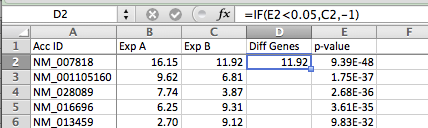# HOMER

Software for motif discovery and next-gen sequencing analysis

## Mastering Excel for Genomics Analysis

Excel is a useful spreadsheet program that is used in nearly every research laboratory (and pretty much every workplace that uses computers).  Excel can be very useful for the following functions:
• Data manipulation and analysis
• Data visualization, creation of graphs/figures
• Formatting of data for use in other programs
Excel is not the best program available for any of the tasks above, but it is the most practical tool sometimes based on it's cheap and ubiquitous.  Also, since there are approximately 20,000-30,000 genes, this fits nicely into the upper limits of what Excel can handle.

### Basic Tips for Excel:

• When highlighting a selection, NEVER drag!  Hold Shift+Ctrl+Arrow Key to auto select to the end of the worksheet, or until there is a blank space.
• To auto-complete a formula, double-click on the little black box on the low-right of an active cell.
• Always use 'normal view' (i.e. menu bar->View->Normal).  Don't use 'Page Layout' unless you have a good reason.
• Consider making Excel the default program for files with extensions *.txt, *.csv, *.tsv, etc.  (can save a lot of time instead of using "open with Excel...")

### Highlighting Differential Genes in a Graph

X-Y scatter plots are a great way to display information for figures in papers or presentations - much much better than venn diagrams, which should probably be outlawed.  Often you may want to highlight some of the point in the plot to draw attention to them - for example, highlight the points that represent differentially expressed genes.

The general strategy I recommend is to plot a second set of points 'over' the first set.  You can use an 'if' statement formula to determine if the gene is differentially expressed based on a p-value or fold change: if the data point is differentially expressed, plot the data over the original data point.  If not, plot the data point 'off' the graph where you cannot see it.

1. Load you data in to excel.  Lets assume you have log-transformed expression values for two experiments and a p-value for each gene.  For example:2. Insert a new column to the right of the columns you want to plot (in this case between C & D).  Put "Diff Genes" or some type of description in the first row of the new column
3. Enter the formula =IF(1*,2*,3*) in the 2nd row of the new column, where 1*, 2*, and 3* are:
1. Conditional test for differential expression (in example below, E2 < 0.05 for checking if the p-value is less than 0.05)
2. The value if the test is true - we want to value to be the y-value in the x-y plot (C2 in this example)
3. The value if the test is false - we want a value that is 'away from the data' - in this case -1, which will not overlap with any of the data from the first two columns4. Autocomplete the formula down the whole column by selecting the cell with the formula, and then double-clicking on the little black box in the lower right-hand corner of the box.
5. Highlight all 3 data columns (original data from Exp A, Exp B, and the new column with the formula) and create an X-Y scatter plot.6. Now that you've created the plot - now it's time to clean up the setting to make it pretty.  The first thing you want to do is change the y-axis to only show values above zero - to get ride of the red points representing genes that were not differentially expressed (-1 from the IF statement above).  After changing the colors, size of the points, and changing the y-axis, you can get something that looks like this:7.  You can also try updating the formula to change the p-value threshold (i.e. 0.001 instead of 0.05) - change the formula at the top, and then autocomplete it and watch the graph update automatically!

### Adding Gene Annotation (i.e. Database Join in Excel using vlookup):

First thing you need is an annotation table, with the ID you want to convert with the gene name or other information on the same line.

1. Load the ID annotation table into Excel.
3. Make sure the accession/ID that you want to convert is in the far left column of the annotation table.
4. Sort the annotation table by the far left column (the ID you want to convert)
5. Copy the annotation table next to you data in the same spreadsheet (you can leave a column of space to keep them separate)
6. Insert a new column into YOUR data next the ID you want to convert.
7. Enter the formula for =VLOOKUP(1*, 2*, 3*, 4*), where 1*, 2*, 3*, and 4* are:
1. lookup value (the cell of the ID you want to convert in your data table)
2. the entire ID annotation table.  Highlight the whole thing (no header).  VERY IMPORTANT - after you highlight the whole thing, add "\$" in front of the row and column values. For example: K2:O21344 >> \$K\$2:\$O\$21344.   This way, when you auto-complete or drag the formula, these values will not change
3. Enter the column number, in the annotation table, that you want to return (i.e. "2").
4. Enter "FALSE" - you never want to enter true for this value.
8. Auto-complete the formula down the length of your data.
9. Copy the new values and then paste-special the "values" to remove the formula
10. Delete the annotation table - and you're done!Can't figure something out? Questions, comments, concerns, or other feedback:
cbenner@salk.edu